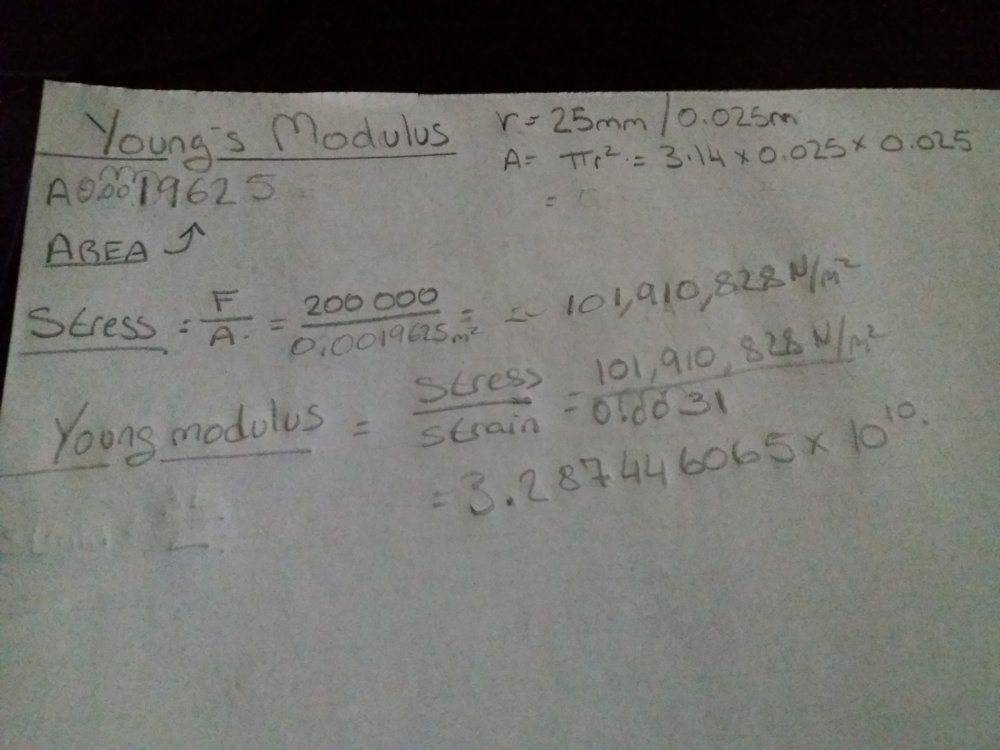# Young's modulus

Homework Statement:
What s wrong if there is something wrong .
Relevant Equations:
Stress over strainSome one knows if its ok like this the answers?

haruspex
Homework Helper
Gold Member
2020 Award
Homework Statement:: What s wrong if there is something wrong .
Relevant Equations:: Stress over strain

View attachment 256819
Some one knows if its ok like this the answers?
Hard to say since you do not state the actual question. Inferring the question from your working, it looks right except that you quote far too many digits. The data you are given are only to two significant figures, so that's how your answer should be.

•chriscarson
So must be 3.2 x 10 to the power of 18 ?

haruspex
Homework Helper
Gold Member
2020 Award
So must be 3.2 x 10 to the power of 18 ?
No, 3.28... would round to 3.3, not 3.2. And where did the 18 come from? You had 1010.

•chriscarson
Hard to say since you do not state the actual question. Inferring the question from your working, it looks right except that you quote far too many digits. The data you are given are only to two significant figures, so that's how your answer should be.

So it have to be only 3.2 ?

No, 3.28... would round to 3.3, not 3.2. And where did the 18 come from? You had 1010.

Oh yes sorry , to the power of 10 .
Look s like everytime I Work a sum everytime different answer .
Thanks